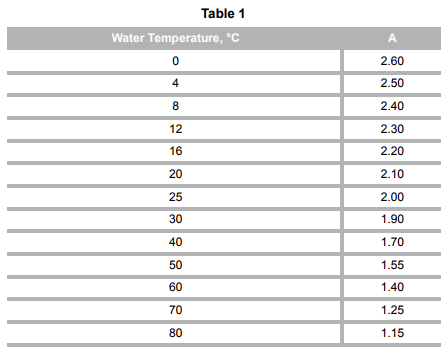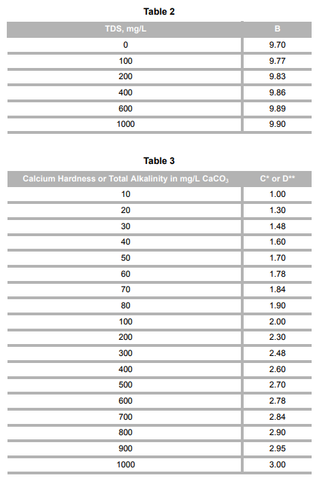###### February 20, 2018# Langelier Saturation Index

The Langelier Saturation Index (LI), a measure of a solution’s ability to dissolve

or deposit calcium carbonate, is often used as an indicator of the corrosivity of

water. The index is not related directly to corrosion, but is related to the

deposition of a calcium carbonate film or scale; this covering can insulate pipes,

boilers, and other components of a system from contact with water. When no

protective scale is formed, water is considered to be aggressive, and corrosion

can occur. Highly corrosive water can cause system failures or result in health

problems because of dissolved lead and other heavy metals. An excess of scale

can also damage water systems, necessitating repair or replacement.

In developing the LI, Langelier derived an equation for the pH at which water is

saturated with calcium carbonate (pHs). This equation is based on the

equilibrium expressions for calcium carbonate solubility and bicarbonate

dissociation. To approximate actual conditions more closely, pHs calculations

were modified to include the effects of temperature and ionic strength.

The Langelier Index is defined as the difference between actual pH (measured)

and calculated pHs. The magnitude and sign of the LI value show water’s

tendency to form or dissolve scale, and thus to inhibit or encourage corrosion.

Although information obtained from the LI is not quantitative, it can be useful in

estimating water treatment requirements for low pressure boilers, cooling

towers, and water treatment plants, as well as serving as a general indicator of

the corrosivity of water.

Parameter Measurement

The Langelier Saturation Index can be calculated easily by using Hach products

to determine the pH of calcium carbonate saturation (pH) and the actual pH of a

solution. Using a simple formula, the pH is derived from the values for calcium

hardness, total alkalinity at pH 4.5, temperature, and total filterable residue

(total dissolved solids). All of these procedures are included in this manual.

Temperature:

Temperature can be measured in degrees celsius with a laboratory thermometer

(Cat. No. 566-01). If only a Fahrenheit thermometer is available, conversion to

degrees Celsius will be necessary.

[°C = 5/9 x (°F – 32)].

Calculation

After the preceding parameters have been determined, calculate the pH from the

following formula:

Where:

Constant A takes into account the effect of temperature. It is found by selecting

the value from Table 1 on page 3 that corresponds to the measured temperature

in degrees Celsius.

Constant B is a correction for the ionic strength of the sample. It is determined

using Table 2 on page 4 by taking the value that corresponds to the measured

total filterable residue or the estimated total dissolved solids (TDS).

pHs = A B C – D + –

Value C is obtained from Table 3 on page 4 by reading the value corresponding to

the calcium hardness (in mg/L CaCO3) of the sample.

Value D is obtained from Table 3 by reading the measured value for total

alkalinity (in mg/L CaCO3) of the sample.

The Langelier Saturation Index is the difference between the actual pH of the

solution and pHs calculated above.

Interpretation

The LI is a gauge of whether a water will precipitate or dissolve calcium

carbonate. If the pHs is equal to the actual pH, the water is considered

“balanced”. This means that calcium carbonate will not be dissolved or

precipitated. If the pHs is less than the actual pH (the LI is a positive number),

the water will tend to deposit calcium carbonate and is scale-forming

(nonaggressive). If the pHs is greater than the actual pH (the LI is a negative

number), the water is not saturated and will dissolve calcium carbonate

(aggressive). In summary:

pHS = pHactual, water is balanced

pHS < pHactual, water is scale forming (nonaggressive)

pHS > pHactual, water is not scale forming (aggressive)

It is important to remember that the LI value is not a quantitative measure of

calcium carbonate saturation or corrosion.

Because the protective scale formation is dependent on pH, bicarbonate ion,

calcium carbonate, dissolved solids, and temperature; each may affect the

water’s corrosive tendencies independently. Soft, low-alkalinity waters with

either low or excessively high pH are corrosive, even though this may not be

predicted by the LI. This is because insufficient amounts of calcium carbonate

and alkalinity are available to form a protective scale.

Waters with high pH values and sufficient hardness and alkalinity may also be

corrosive, even if the LI predicts the opposite. This is the result of calcium and

magnesium complexes that cannot actively participate in the scale forming

process. Analytical procedures do not distinguish between these complexes and

available calcium and magnesium; therefore, the LI value is not accurate in such

situations.

Corrosive tendencies may also be exhibited by water containing high

concentrations of sulfate, chloride, and other ions which interfere with uniform

carbonate film formation.

As a result of these and other problems, the LI is useful only for determining the

corrosivity of waters containing more than 40 mg/L of alkalinity, sufficient

calcium ion concentration, and ranging between pH 6.5 and 9.5.

LI pHactual pHs = –

Aggressive Index

The Aggressive Index (AI), originally developed for monitoring water in

asbestos pipe, is sometimes substituted for the Langelier Index as an indicator of

the corrosivity of water. The AI is derived from the actual pH, calcium hardness,

and total alkalinity. (Use procedures contained in this handbook). Where it is

applicable, it is simpler and more convenient than the LI. Because the AI does

not include the effects of temperature or dissolved solids, it is less accurate as an

analytical tool than the LI.

Calculation

After obtaining the pH, total alkalinity, and calcium hardness, use the following

formula to calculate the AI:

Al = pHactual + C + D

Where:

Value C is obtained from Table 3 on page 4 by reading the value corresponding to

the calcium hardness (in mg/L CaCO3) of the sample.

Value D is obtained from Table 3 by reading the measured value for total

alkalinity (in mg/L CaCO3) of the sample.

Interpretation

As with LI, the AI is not a quantitative measure of corrosion, but is a general

indicator of the tendency for corrosion to occur, and as such, should be used

with proper reservation. An AI of 12 or above indicates nonaggressive (not

corrosive) water. AI values below 10 indicate extremely aggressive (corrosive)

conditions. Values of 10–11.9 suggest that the water is moderately aggressive.

Corrosivity characteristics of water as indicated by the LI and AI are compared

in Table 4.* Factor C is the logarithm (base 10) of the calcium hardness expressed in mg/L

** Factor D is the logarithm (base 10) of the total alkalinity expressed in mg/L

Table 4Corrosive Characteristics Langelier Index Aggressive Index

Highly aggressive < –2.0 < 10.0

Moderately aggressive –2.0 to 0.0 10.00 to 12.0

Nonaggressive >0.0 >12.0

LSI Calculator: https://www.lenntech.com/calculators/langelier/index/langelier.htm###### Aldo Zaffalon

Author

Water treatment automation expert, with over 25 years of experience in industrial water treatment automation, marketing, sales and engineering.

### Related Posts

#### 2 Responses###### Aldo Zaffalon

August 26, 2019

Unfortunately there is not a one device that measures and calculates LSI, as far as we know. Some variables are easy to measure, like temperature and pH. Other, like Alkalinity, and Hardness, require complex instruments. Also, the LSI will not change very fast in a cooling tower so normally, when water analysis is performed, a quick calculation of LSI can be performed. If you wish, please send us an email to sales@yamathosupply.com. We can work some equipment with some of our suppliers.###### Thabit Abbas

August 26, 2019

Dear Experts
I wonder if you could supply us with online integrated Langelier Index .(direct dial reading in the cooling tower )
thank you
Thabit Abbas /Iraq